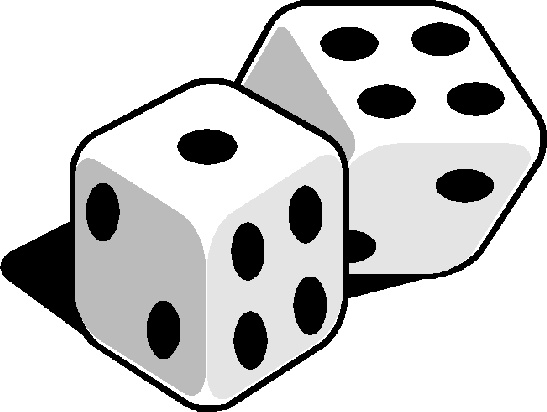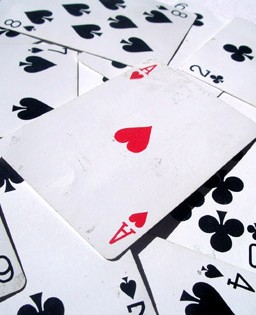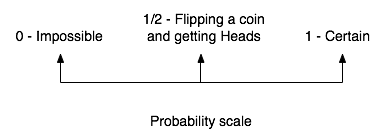# Numb3rs 212: The OG

Don and his team are called to the scene of the murder of a Los Angeles gang member, but they soon learn that they are investigating the murder of another agent who had been working undercover.

Amongst other techniques, Charlie uses Probability Theory and Poisson Distributions to help better understand the L.A. gang scene.## Probability 101

The scientific study of probability is a modern development, but gambling shows that there has been an interest in quantifying the ideas of probability for millennia. The exact mathematical descriptions of use in those problems only arose much later though. For more historical background, check this webpage.Chance is a natural and intuitive concept, and one can easily make and understand sentences like "the chances of you running a mile in less than 20 seconds are less than those of you rolling a 7 with two ordinary dice". Probability is the branch of mathematics that makes it possible to quantify, compare and study such statments, by assigning to an event such as "flipping a coin and showing heads" a number between 0 and 1, called its probability (of occuring), where 0 means that it is (almost) impossible for the event to occur and 1 means it is (almost) certain, while 1/2 means that if you try the experiment a million times, about half of the tries will lead to a positive outcome, while the other half will lead to a negative one.### Probability as proportion

In its most basic form, to define the probability of an event, one usually proceeds as follows:

1. First of all, we need a random experiment, also called the universe, such as "flipping an ordinary coin" or "rolling two ordinary dice". Mathematically, this is defined by listing all the things that can happen that we are interested in. In doing that, we make sure we only list the basic outcomes that are not a combination of other ones, and we also make sure all the outcomes we have are equally likely to happen.
For example, we would list {Heads, Tails} for the first example, and all the die combinations {(1,1), (1, 2), ... , (3, 4), (3, 5), ..., (6, 6)} for the second one, where (m, n) means the first die rolled an m and the second one rolled an n. If the coin is tempered with so that it falls on Heads twice as often as it falls on Tails, then we can't use {Heads, Tails} anymore. We'll see in the next section of to deal with these by introducing random variables.
2. Then we need an event whose probability we want to compute, for example "rolling a 6" in the two dice example. Mathematically, this amounts to looking at the basic outcomes in the universe and listing out all those that realize our event.
In the two dice example, "rolling a 6" is realized by combinations like (1, 5), (2, 4) and (3, 3). Are there any other ones?
3. Finally, the probability of such an event is then defined as follows:Some examples!
Towards Conditional Probability

### Random variables

The Bernoulli random variable

## The Poisson Distribution: **need to list ref/source for the math derivation

Counting occurences of rare events is a very common problem that arises in almost every branch of science. Here are but a couple of examples of such situations.

1. Meteorites. The Earth is bombarded by an endless shower of meteorites. Rarely, they hit the ground. It is natural to count how many meteorite-strikes there are on patch of ground during a fixed period. (For example, on your house, while you are living there.)
2. Accidents. Any stretch of road, or road junction, is subject to the occasional accident. How any are there in a given stretch of road? How many are there during a fixed period at some intersection?
3. Business phone calls, received by Pam on a given day at the Scranton Dunder Mifflin branch.

Clearly, this is a list which could be extended indefinitely. You have to think only for a moment of the applications to counting: colonies of bacteria on a dish, flaws in a carpet, bugs in a program, photons in your telescope, lighning strikes on your steeple, wasps in your beer, and so on.
In order to study the mathematics behind this problem, let us focus on one of those examples which will represent or act as a model for all the rest. Let us count the number of phone calls received by Pam, during a time period of length t, [0, t] say.

The period is divided up into n equal intervals; as we make the intervals smaller (weeks, days, seconds, ...), the number n becomes larger. We assume that the intervals are so small that the chance of two or more shootings in the same intervalis negligible.

Furthermore, different clients take no account of other clients' calendars, in the sense that it is reasonable to suppose that phone calls in different intervals are independent, and that the chance of a phone call is the same for each of the n intervals, p say. (A more advanced model would take into account the fact that phone calls sometimes come in showers.) Thus the total number of calls in the n intervals is the same as the number of successes in n Bernoulli trials, with distributionwhich is binomial. These assumptions are in fact well supported by observation.

Now obviously p depends on the size of the interval; there must be more chance of a call during a month than during a second. Also it seems reasonable that if p is the chance of call in one minute, then the chance of call in two minutes should be 2p, and so on. This amounts to the assumption that n*p/t is a constant, which we call lambda. So n*p = lambda*t

Thus as we increase n and decrease p so that lambda * t is fixed, we have the following situation: *****

Derive the poisson distribution

The important point about the aboce derivation is that it is generally applicable to many other similar circumstances. Thus, for example, we could replace 'phone calls' by 'chocalate chips' and 'the interval [0,t]' by 'a chocolate cake'; the 'n divisions of the interval' then become 'the n slices of the cake', and we may find that a chocolate cake made from a large batch of mixed dough will contain a number chocolate chips with a Poisson distribution, approximately.

The same arguments has yielded approximate Poisson distributions observed for flying bomb hits on London in 1939-45, soldiers disabled by horse-kicks in the Prussian Cavalry, accidents along a stretch of road, and so on. In general, rare events that occur independently but consistently in some region of time or spacem or both, will often follow a Poisson distribution. For this reason, it is often called the law of rare events.

Notice that we have to count events that are isolated, that is to say occur singly, because we have assumed that only one event is possible in a short enough interval. Therfore we do not expect the number of people involved in accidents at a junction to have a simple Poisson distribution, because there may be several in each vehicle. Likewise, the number of daisy flowers in your lawn may not be Poisson, because each plant has a cluster of flowers. And the number of bacteria on a Petri dish may not be Poisson, because the separate colonies form tightly groups. The colonies, however, may well have an approximate Poisson distribution.

Activity
1. A cook adds 200 chocolate chips to a batch of dough, and makes 40 biscuits. What is the appoximate value of the probability that a random biscuit has at least 4 chips? no chips at all?
2. A jumbo jet carries 400 passengers. Any passenger indpenently fails to show up with probability 10-2. If the airline makes 404 reservations, what is the probability that it has to bump at least one passenger?
3. Find the mode of the Poisson distribution. Is it always unique?